## Cutting Speed (vc)

Check the item you want to calculate, input values in the two boxes, and then press the Calculate button.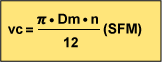vc (SFM) : Cutting Speed
Dm (Inch) : Work Material Diameter
π (3.14) : Pi
n (min-1) : Main Axis Spindle Speed

n(min-1)
Dm(inch)
vc(SFM)

### Problem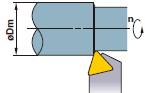What is the cutting speed when main axis spindle speed is 700min-1and external diameter is Φ2 ?

Substitute π=3.14, Dm=2, n=700 into the formulae.
vc=(π×Dm×n)÷12=(3.14×2×700)÷12
=366.33SFM

Cutting speed is 366.33SFM.

## Feed (f)

Check the item you want to calculate, input values in the two boxes, and then press the Calculate button.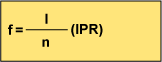f (IPR) : Feed per Revolution
I (IPM) : Cutting Length per Min.
n (min-1) : Main Axis Spindle Speed

I (IPM)
n(min-1)
f(IPR)

### ProblemWhat is the feed per revolution when main axis spindle speed is 500min-1 and cutting length per minute is 120mm/min ?

Substitute n=500, I=4.72 into the formulae.
f=l÷n=4.72÷500=.009IPR

## Cutting time (Tc)

Input the values.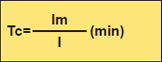Tc(min):Cutting Time
lm(Inch):Work Material Length
I (IPM):Cutting Length per Min.

lm(inch)
l(IPM)
Tc(min)
second(s)

### Problem

What is the cutting time when 4inch work material is machined at 1000min-1 with feed = .008IPR ?

First, calculate the cutting length per min. from the feed and spindle speed.
l=f×n=.008×1000=8IPM
Substitute the answer above into the formulae.
Tc=lm÷l=4÷8=0.5(min)
0.5×60=30(sec) The answer is 30 sec.

## Theoretical finished surface roughness (h)

Input the values.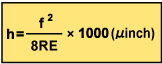h (µinch):Finished Surface Roughness
f (IPR):Feed per Revolution

f(IPR)
RE(inch)
h(µinch）

### Problem

What is the theoretical finished surface roughness when the insert corner radius is .031inch and feed is .008 IPR ?

Substitute f=.008IPR, RE=.031 into the formulae.
h=(.008)2÷(8×.031)×1000=.258µinch
The theoretical finished surface roughness is .258µinch.

## Cutting Speed (vc)

Check the item you want to calculate, input values in the two boxes, and then press the Calculate button.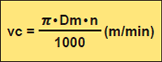※Divide by 1000 to change to m from mm.
vc (m/min) : Cutting Speed
Dm (mm) : Workpiece Diameter
π (3.14) : Pi
n (min-1) : Main Axis Spindle Speed

n(min-1)
Dm(mm)
vc(m/min)

### ExampleWhat is the cutting speed when main axis spindle speed is 700min-1and external diameter is Φ50 ?

Substitute π=3.14, Dm=50, n=700 into the formula.
vc=(π×Dm×n)÷1000=(3.14×50×700)÷1000
=110(m/min)

Cutting speed is 110m/min.

## Feed (f)

Check the item you want to calculate, input values in the two boxes, and then press the Calculate button.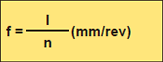f (mm/rev) : Feed per Revolution
I (mm/min) : Cutting Length per Min.
n (min-1) : Main Axis Spindle Speed

l(mm/min)
n(min-1)
f(mm/rev)

### ExampleWhat is the feed per revolution when main axis spindle speed is 500min-1 and cutting length per minute is 120mm/min ?

Substitute n=500, I=120 into the formula.
f=l÷n=120÷500=0.24(mm/rev)

## Cutting time (Tc)

Input the values.Tc(min):Cutting Time
lm(mm):Workpiece Length
ｌ(mm/min):Cutting Length per Min.

lm(mm)
l(mm/min)
Tc(min)
second(s)

### Example

What is the cutting time when 100mm workpiece is machined at 1000min-1 with feed = 0.2mm/rev ?

First, calculate the cutting length per min. from the feed and spindle speed.
l=f×n=0.2×1000=200(mm/min)
Substitute the answer above into the formula.
Tc=lm÷l=100÷200=0.5(min)

## Theoretical finished surface roughness (h)

Input the values.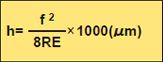h (µm):Finished Surface Roughness
f (mm/rev):Feed per Revolution

f(mm/rev)
RE(mm)
h(μm）

### ExampleWhat is the theoretical finished surface roughness when the insert corner radius is 0.8mm and feed is 0.2mm/rev ?